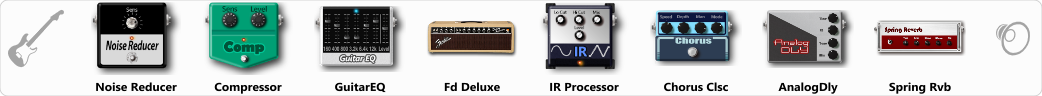# The Blues Sound

Discussion in 'ToneLib-GFX presets' started by ktaxi, Jul 31, 2020 at 1:18 PM.

1. The Blues Sound

Preset name: The Blues Sound

Bridge pickup,i hope you like it!!!

Effects chain:Effect: "Noise Reducer" (Dynamics / Filter), active - "yes"
"Sens" = 15
"Mode" = Soft

Effect: "Compressor" (Dynamics / Filter), active - "yes"
"Sense" = 38
"Level" = 36

Effect: "GuitarEQ" (Dynamics / Filter), active - "yes"
"160 Hz" = 0
"400 Hz" = 1
"800 Hz" = 2
"1.6 kHz" = 4
"3.2 kHz" = 3
"6.4 kHz" = 2
"12 kHz" = 1
"Level (dB)" = 0

Effect: "Fd Deluxe" (Amp simulators), active - "yes"
"Gain" = 58
"Bass" = 41
"Middle" = 100
"Treble" = 66
"Presence" = 64
"Master" = 100
"Output" = 50
"Level (dB)" = 5

Effect: "IR Processor" (Dynamics / Filter), active - "yes"
"IR" = Fender Champ 57 On Axis, 1 in
"Low Cut (Hz)" = 0
"Hi Cut (kHz)" = 20.0
"Mix" = 100
"Level (dB)" = 0

Effect: "Chorus Clsc" (Modulation / Sfx), active - "yes"
"Speed" = 2.4
"Depth" = 33
"Center" = 2.6
"Mode" = Vibrato

Effect: "AnalogDly" (Delay), active - "yes"
"Time" = 380
"Feedback" = 18
"Tone" = 56
"Mix" = 35

Effect: "Spring Rvb" (Reverb), active - "yes"
"Time" = 4.9
"PreDelay" = 0
"LoDamp" = 16
"HiDamp" = 26
"Mix" = 37

Note: You will need to download and install the ToneLib-GFX software to use the preset.

#### Attached Files:

• ###### The_Blues_Sound.tlgfx
File size:
4.4 KB
Views:
201
Alexey Borovkov and steveisall like this.
2. steveisall thanks!!!

3. thank you Alexey Borovkov!!!